# P-99: Ninety-Nine Prolog Problems

#### werner.hett@bfh.ch or werner.hett@gmail.com

The purpose of this problem collection is to give you the opportunity to practice your skills in logic programming. Your goal should be to find the most elegant solution of the given problems. Efficiency is important, but logical clarity is even more crucial. Some of the (easy) problems can be trivially solved using built-in predicates. However, in these cases, you learn more if you try to find your own solution.

Every predicate that you write should begin with a comment that describes the predicate in a declarative statement. Do not describe procedurally, what the predicate does, but write down a logical statement which includes the arguments of the predicate. You should also indicate the intended data types of the arguments and the allowed flow patterns.

The problems have different levels of difficulty. Those marked with a single asterisk (*) are easy. If you have successfully solved the preceeding problems you should be able to solve them within a few (say 15) minutes. Problems marked with two asterisks (**) are of intermediate difficulty. If you are a skilled Prolog programmer it shouldn't take you more than 30-90 minutes to solve them. Problems marked with three asterisks (***) are more difficult. You may need more time (i.e. a few hours or more) to find a good solution.

## Working with Prolog lists

A list is either empty or it is composed of a first element (head) and a tail, which is a list itself. In Prolog we represent the empty list by the atom [] and a non-empty list by a term [H|T] where H denotes the head and T denotes the tail.

P01 (*) Find the last element of a list.
Example:
?- my_last(X,[a,b,c,d]).
X = d

P02 (*) Find the last but one element of a list.
(zweitletztes Element, l'avant-dernier �l�ment)

P03 (*) Find the K'th element of a list.
The first element in the list is number 1.
Example:
?- element_at(X,[a,b,c,d,e],3).
X = c

P04 (*) Find the number of elements of a list.

P05 (*) Reverse a list.

P06 (*) Find out whether a list is a palindrome.
A palindrome can be read forward or backward; e.g. [x,a,m,a,x].

P07 (**) Flatten a nested list structure.
Transform a list, possibly holding lists as elements into a `flat' list by replacing each list with its elements (recursively).

Example:
?- my_flatten([a, [b, [c, d], e]], X).
X = [a, b, c, d, e]

Hint: Use the predefined predicates is_list/1 and append/3

P08 (**) Eliminate consecutive duplicates of list elements.
If a list contains repeated elements they should be replaced with a single copy of the element. The order of the elements should not be changed.

Example:
?- compress([a,a,a,a,b,c,c,a,a,d,e,e,e,e],X).
X = [a,b,c,a,d,e]

P09 (**) Pack consecutive duplicates of list elements into sublists.
If a list contains repeated elements they should be placed in separate sublists.

Example:
?- pack([a,a,a,a,b,c,c,a,a,d,e,e,e,e],X).
X = [[a,a,a,a],[b],[c,c],[a,a],[d],[e,e,e,e]]

P10 (*) Run-length encoding of a list.
Use the result of problem P09 to implement the so-called run-length encoding data compression method. Consecutive duplicates of elements are encoded as terms [N,E] where N is the number of duplicates of the element E.

Example:
?- encode([a,a,a,a,b,c,c,a,a,d,e,e,e,e],X).
X = [[4,a],[1,b],[2,c],[2,a],[1,d][4,e]]

P11 (*) Modified run-length encoding.
Modify the result of problem P10 in such a way that if an element has no duplicates it is simply copied into the result list. Only elements with duplicates are transferred as [N,E] terms.

Example:
?- encode_modified([a,a,a,a,b,c,c,a,a,d,e,e,e,e],X).
X = [[4,a],b,[2,c],[2,a],d,[4,e]]

P12 (**) Decode a run-length encoded list.
Given a run-length code list generated as specified in problem P11. Construct its uncompressed version.

P13 (**) Run-length encoding of a list (direct solution).
Implement the so-called run-length encoding data compression method directly. I.e. don't explicitly create the sublists containing the duplicates, as in problem P09, but only count them. As in problem P11, simplify the result list by replacing the singleton terms [1,X] by X.

Example:
?- encode_direct([a,a,a,a,b,c,c,a,a,d,e,e,e,e],X).
X = [[4,a],b,[2,c],[2,a],d,[4,e]]

P14 (*) Duplicate the elements of a list.
Example:
?- dupli([a,b,c,c,d],X).
X = [a,a,b,b,c,c,c,c,d,d]

P15 (**) Duplicate the elements of a list a given number of times.
Example:
?- dupli([a,b,c],3,X).
X = [a,a,a,b,b,b,c,c,c]

What are the results of the goal:
?- dupli(X,3,Y).

P16 (**) Drop every N'th element from a list.
Example:
?- drop([a,b,c,d,e,f,g,h,i,k],3,X).
X = [a,b,d,e,g,h,k]

P17 (*) Split a list into two parts; the length of the first part is given.
Do not use any predefined predicates.

Example:
?- split([a,b,c,d,e,f,g,h,i,k],3,L1,L2).
L1 = [a,b,c]
L2 = [d,e,f,g,h,i,k]

P18 (**) Extract a slice from a list.
Given two indices, I and K, the slice is the list containing the elements between the I'th and K'th element of the original list (both limits included). Start counting the elements with 1.

Example:
?- slice([a,b,c,d,e,f,g,h,i,k],3,7,L).
X = [c,d,e,f,g]

P19 (**) Rotate a list N places to the left.
Examples:
?- rotate([a,b,c,d,e,f,g,h],3,X).
X = [d,e,f,g,h,a,b,c]

?- rotate([a,b,c,d,e,f,g,h],-2,X).
X = [g,h,a,b,c,d,e,f]

Hint: Use the predefined predicates length/2 and append/3, as well as the result of problem P17.

P20 (*) Remove the K'th element from a list.
Example:
?- remove_at(X,[a,b,c,d],2,R).
X = b
R = [a,c,d]

P21 (*) Insert an element at a given position into a list.
Example:
?- insert_at(alfa,[a,b,c,d],2,L).
L = [a,alfa,b,c,d]

P22 (*) Create a list containing all integers within a given range.
Example:
?- range(4,9,L).
L = [4,5,6,7,8,9]

P23 (**) Extract a given number of randomly selected elements from a list.
The selected items shall be put into a result list.
Example:
?- rnd_select([a,b,c,d,e,f,g,h],3,L).
L = [e,d,a]

Hint: Use the built-in random number generator random/2 and the result of problem P20.

P24 (*) Lotto: Draw N different random numbers from the set 1..M.
The selected numbers shall be put into a result list.
Example:
?- rnd_select(6,49,L).
L = [23,1,17,33,21,37]

Hint: Combine the solutions of problems P22 and P23.

P25 (*) Generate a random permutation of the elements of a list.
Example:
?- rnd_permu([a,b,c,d,e,f],L).
L = [b,a,d,c,e,f]

Hint: Use the solution of problem P23.

P26 (**) Generate the combinations of K distinct objects chosen from the N elements of a list
In how many ways can a committee of 3 be chosen from a group of 12 people? We all know that there are C(12,3) = 220 possibilities (C(N,K) denotes the well-known binomial coefficients). For pure mathematicians, this result may be great. But we want to really generate all the possibilities (via backtracking).

Example:
?- combination(3,[a,b,c,d,e,f],L).
L = [a,b,c] ;
L = [a,b,d] ;
L = [a,b,e] ;
...

P27 (**) Group the elements of a set into disjoint subsets.
a) In how many ways can a group of 9 people work in 3 disjoint subgroups of 2, 3 and 4 persons? Write a predicate that generates all the possibilities via backtracking.

Example:
?- group3([aldo,beat,carla,david,evi,flip,gary,hugo,ida],G1,G2,G3).
G1 = [aldo,beat], G2 = [carla,david,evi], G3 = [flip,gary,hugo,ida]
...

b) Generalize the above predicate in a way that we can specify a list of group sizes and the predicate will return a list of groups.

Example:
?- group([aldo,beat,carla,david,evi,flip,gary,hugo,ida],[2,2,5],Gs).
Gs = [[aldo,beat],[carla,david],[evi,flip,gary,hugo,ida]]
...

Note that we do not want permutations of the group members; i.e. [[aldo,beat],...] is the same solution as [[beat,aldo],...]. However, we make a difference between [[aldo,beat],[carla,david],...] and [[carla,david],[aldo,beat],...].

You may find more about this combinatorial problem in a good book on discrete mathematics under the term "multinomial coefficients".

P28 (**) Sorting a list of lists according to length of sublists
a) We suppose that a list (InList) contains elements that are lists themselves. The objective is to sort the elements of InList according to their length. E.g. short lists first, longer lists later, or vice versa.

Example:
?- lsort([[a,b,c],[d,e],[f,g,h],[d,e],[i,j,k,l],[m,n],[o]],L).
L = [[o], [d, e], [d, e], [m, n], [a, b, c], [f, g, h], [i, j, k, l]]

b) Again, we suppose that a list (InList) contains elements that are lists themselves. But this time the objective is to sort the elements of InList according to their length frequency; i.e. in the default, where sorting is done ascendingly, lists with rare lengths are placed first, others with a more frequent length come later.

Example:
?- lfsort([[a,b,c],[d,e],[f,g,h],[d,e],[i,j,k,l],[m,n],[o]],L).
L = [[i, j, k, l], [o], [a, b, c], [f, g, h], [d, e], [d, e], [m, n]]

Note that in the above example, the first two lists in the result L have length 4 and 1, both lengths appear just once. The third and forth list have length 3 which appears, there are two list of this length. And finally, the last three lists have length 2. This is the most frequent length.

## Arithmetic

P31 (**) Determine whether a given integer number is prime.
Example:
?- is_prime(7).
Yes

P32 (**) Determine the greatest common divisor of two positive integer numbers.
Use Euclid's algorithm.
Example:
?- gcd(36, 63, G).
G = 9

Define gcd as an arithmetic function; so you can use it like this:
?- G is gcd(36,63).
G = 9

P33 (*) Determine whether two positive integer numbers are coprime.
Two numbers are coprime if their greatest common divisor equals 1.
Example:
?- coprime(35, 64).
Yes

P34 (**) Calculate Euler's totient function phi(m).
Euler's so-called totient function phi(m) is defined as the number of positive integers r (1 <= r < m) that are coprime to m.

Example: m = 10: r = 1,3,7,9; thus phi(m) = 4. Note the special case: phi(1) = 1.

?- Phi is totient_phi(10).
Phi = 4

Find out what the value of phi(m) is if m is a prime number. Euler's totient function plays an important role in one of the most widely used public key cryptography methods (RSA). In this exercise you should use the most primitive method to calculate this function (there are smarter ways that we shall discuss later).

P35 (**) Determine the prime factors of a given positive integer.
Construct a flat list containing the prime factors in ascending order.
Example:
?- prime_factors(315, L).
L = [3,3,5,7]

P36 (**) Determine the prime factors of a given positive integer (2).
Construct a list containing the prime factors and their multiplicity.
Example:
?- prime_factors_mult(315, L).
L = [[3,2],[5,1],[7,1]]

Hint: The problem is similar to problem P13.

P37 (**) Calculate Euler's totient function phi(m) (improved).
See problem P34 for the definition of Euler's totient function. If the list of the prime factors of a number m is known in the form of problem P36 then the function phi(m) can be efficiently calculated as follows: Let [[p1,m1],[p2,m2],[p3,m3],...] be the list of prime factors (and their multiplicities) of a given number m. Then phi(m) can be calculated with the following formula:

phi(m) = (p1 - 1) * p1**(m1 - 1) * (p2 - 1) * p2**(m2 - 1) * (p3 - 1) * p3**(m3 - 1) * ...

Note that a**b stands for the b'th power of a.

P38 (*) Compare the two methods of calculating Euler's totient function.
Use the solutions of problems P34 and P37 to compare the algorithms. Take the number of logical inferences as a measure for efficiency. Try to calculate phi(10090) as an example.

P39 (*) A list of prime numbers.
Given a range of integers by its lower and upper limit, construct a list of all prime numbers in that range.

P40 (**) Goldbach's conjecture.
Goldbach's conjecture says that every positive even number greater than 2 is the sum of two prime numbers. Example: 28 = 5 + 23. It is one of the most famous facts in number theory that has not been proved to be correct in the general case. It has been numerically confirmed up to very large numbers (much larger than we can go with our Prolog system). Write a predicate to find the two prime numbers that sum up to a given even integer.

Example:
?- goldbach(28, L).
L = [5,23]

P41 (**) A list of Goldbach compositions.
Given a range of integers by its lower and upper limit, print a list of all even numbers and their Goldbach composition.

Example:
?- goldbach_list(9,20).
10 = 3 + 7
12 = 5 + 7
14 = 3 + 11
16 = 3 + 13
18 = 5 + 13
20 = 3 + 17

In most cases, if an even number is written as the sum of two prime numbers, one of them is very small. Very rarely, the primes are both bigger than say 50. Try to find out how many such cases there are in the range 2..3000.

Example (for a print limit of 50):
?- goldbach_list(1,2000,50).
992 = 73 + 919
1382 = 61 + 1321
1856 = 67 + 1789
1928 = 61 + 1867

## Logic and Codes

P46 (**) Truth tables for logical expressions.
Define predicates and/2, or/2, nand/2, nor/2, xor/2, impl/2 and equ/2 (for logical equivalence) which succeed or fail according to the result of their respective operations; e.g. and(A,B) will succeed, if and only if both A and B succeed. Note that A and B can be Prolog goals (not only the constants true and fail).

A logical expression in two variables can then be written in prefix notation, as in the following example: and(or(A,B),nand(A,B)).

Now, write a predicate table/3 which prints the truth table of a given logical expression in two variables.

Example:
?- table(A,B,and(A,or(A,B))).
true true true
true fail true
fail true fail
fail fail fail

P47 (*) Truth tables for logical expressions (2).
Continue problem P46 by defining and/2, or/2, etc as being operators. This allows to write the logical expression in the more natural way, as in the example: A and (A or not B). Define operator precedence as usual; i.e. as in Java.

Example:
?- table(A,B, A and (A or not B)).
true true true
true fail true
fail true fail
fail fail fail

P48 (**) Truth tables for logical expressions (3).
Generalize problem P47 in such a way that the logical expression may contain any number of logical variables. Define table/2 in a way that table(List,Expr) prints the truth table for the expression Expr, which contains the logical variables enumerated in List.

Example:
?- table([A,B,C], A and (B or C) equ A and B or A and C).
true true true true
true true fail true
true fail true true
true fail fail true
fail true true true
fail true fail true
fail fail true true
fail fail fail true

P49 (**) Gray code.
An n-bit Gray code is a sequence of n-bit strings constructed according to certain rules. For example,
n = 1: C(1) = ['0','1'].
n = 2: C(2) = ['00','01','11','10'].
n = 3: C(3) = ['000','001','011','010',�110�,�111�,�101�,�100�].

Find out the construction rules and write a predicate with the following specification:

% gray(N,C) :- C is the N-bit Gray code

Can you apply the method of "result caching" in order to make the predicate more efficient, when it is to be used repeatedly?

P50 (***) Huffman code.
First of all, consult a good book on discrete mathematics or algorithms for a detailed description of Huffman codes!

We suppose a set of symbols with their frequencies, given as a list of fr(S,F) terms. Example: [fr(a,45),fr(b,13),fr(c,12),fr(d,16),fr(e,9),fr(f,5)]. Our objective is to construct a list hc(S,C) terms, where C is the Huffman code word for the symbol S. In our example, the result could be Hs = [hc(a,'0'), hc(b,'101'), hc(c,'100'), hc(d,'111'), hc(e,'1101'), hc(f,'1100')] [hc(a,'01'),...etc.]. The task shall be performed by the predicate huffman/2 defined as follows:

% huffman(Fs,Hs) :- Hs is the Huffman code table for the frequency table Fs

## Binary Trees

A binary tree is either empty or it is composed of a root element and two successors, which are binary trees themselves.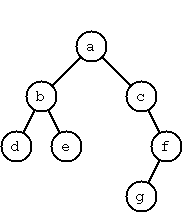In Prolog we represent the empty tree by the atom 'nil' and the non-empty tree by the term t(X,L,R), where X denotes the root node and L and R denote the left and right subtree, respectively. The example tree depicted opposite is therefore represented by the following Prolog term:

T1 = t(a,t(b,t(d,nil,nil),t(e,nil,nil)),t(c,nil,t(f,t(g,nil,nil),nil)))

Other examples are a binary tree that consists of a root node only:

T2 = t(a,nil,nil) or an empty binary tree: T3 = nil

You can check your predicates using these example trees. They are given as test cases in p54.pl.

P54 (*) Check whether a given term represents a binary tree
Write a predicate istree/1 which succeeds if and only if its argument is a Prolog term representing a binary tree.
Example:
?- istree(t(a,t(b,nil,nil),nil)).
Yes
?- istree(t(a,t(b,nil,nil))).
No

P55 (**) Construct completely balanced binary trees
In a completely balanced binary tree, the following property holds for every node: The number of nodes in its left subtree and the number of nodes in its right subtree are almost equal, which means their difference is not greater than one.

Write a predicate cbal_tree/2 to construct completely balanced binary trees for a given number of nodes. The predicate should generate all solutions via backtracking. Put the letter 'x' as information into all nodes of the tree.
Example:
?- cbal_tree(4,T).
T = t(x, t(x, nil, nil), t(x, nil, t(x, nil, nil))) ;
T = t(x, t(x, nil, nil), t(x, t(x, nil, nil), nil)) ;
etc......No

P56 (**) Symmetric binary trees
Let us call a binary tree symmetric if you can draw a vertical line through the root node and then the right subtree is the mirror image of the left subtree. Write a predicate symmetric/1 to check whether a given binary tree is symmetric. Hint: Write a predicate mirror/2 first to check whether one tree is the mirror image of another. We are only interested in the structure, not in the contents of the nodes.

P57 (**) Binary search trees (dictionaries)
Use the predicate add/3, developed in chapter 4 of the course, to write a predicate to construct a binary search tree from a list of integer numbers.
Example:
?- construct([3,2,5,7,1],T).
T = t(3, t(2, t(1, nil, nil), nil), t(5, nil, t(7, nil, nil)))

Then use this predicate to test the solution of the problem P56.
Example:
?- test_symmetric([5,3,18,1,4,12,21]).
Yes
?- test_symmetric([3,2,5,7,4]).
No

Apply the generate-and-test paradigm to construct all symmetric, completely balanced binary trees with a given number of nodes. Example:
?- sym_cbal_trees(5,Ts).
Ts = [t(x, t(x, nil, t(x, nil, nil)), t(x, t(x, nil, nil), nil)), t(x, t(x, t(x, nil, nil), nil), t(x, nil, t(x, nil, nil)))]

How many such trees are there with 57 nodes? Investigate about how many solutions there are for a given number of nodes? What if the number is even? Write an appropriate predicate.

P59 (**) Construct height-balanced binary trees
In a height-balanced binary tree, the following property holds for every node: The height of its left subtree and the height of its right subtree are almost equal, which means their difference is not greater than one.

Write a predicate hbal_tree/2 to construct height-balanced binary trees for a given height. The predicate should generate all solutions via backtracking. Put the letter 'x' as information into all nodes of the tree.
Example:
?- hbal_tree(3,T).
T = t(x, t(x, t(x, nil, nil), t(x, nil, nil)), t(x, t(x, nil, nil), t(x, nil, nil))) ;
T = t(x, t(x, t(x, nil, nil), t(x, nil, nil)), t(x, t(x, nil, nil), nil)) ;
etc......No

P60 (**) Construct height-balanced binary trees with a given number of nodes
Consider a height-balanced binary tree of height H. What is the maximum number of nodes it can contain?
Clearly, MaxN = 2**H - 1. However, what is the minimum number MinN? This question is more difficult. Try to find a recursive statement and turn it into a predicate minNodes/2 defined as follwos:

% minNodes(H,N) :- N is the minimum number of nodes in a height-balanced binary tree of height H.
(integer,integer), (+,?)

On the other hand, we might ask: what is the maximum height H a height-balanced binary tree with N nodes can have?

% maxHeight(N,H) :- H is the maximum height of a height-balanced binary tree with N nodes
(integer,integer), (+,?)

Now, we can attack the main problem: construct all the height-balanced binary trees with a given nuber of nodes.

% hbal_tree_nodes(N,T) :- T is a height-balanced binary tree with N nodes.

Find out how many height-balanced trees exist for N = 15.

P61 (*) Count the leaves of a binary tree
A leaf is a node with no successors. Write a predicate count_leaves/2 to count them.

% count_leaves(T,N) :- the binary tree T has N leaves

P61A (*) Collect the leaves of a binary tree in a list
A leaf is a node with no successors. Write a predicate leaves/2 to collect them in a list.

% leaves(T,S) :- S is the list of all leaves of the binary tree T

P62 (*) Collect the internal nodes of a binary tree in a list
An internal node of a binary tree has either one or two non-empty successors. Write a predicate internals/2 to collect them in a list.

% internals(T,S) :- S is the list of internal nodes of the binary tree T.

P62B (*) Collect the nodes at a given level in a list
A node of a binary tree is at level N if the path from the root to the node has length N-1. The root node is at level 1. Write a predicate atlevel/3 to collect all nodes at a given level in a list.

% atlevel(T,L,S) :- S is the list of nodes of the binary tree T at level L

Using atlevel/3 it is easy to construct a predicate levelorder/2 which creates the level-order sequence of the nodes. However, there are more efficient ways to do that.

P63 (**) Construct a complete binary tree
A complete binary tree with height H is defined as follows: The levels 1,2,3,...,H-1 contain the maximum number of nodes (i.e 2**(i-1) at the level i, note that we start counting the levels from 1 at the root). In level H, which may contain less than the maximum possible number of nodes, all the nodes are "left-adjusted". This means that in a levelorder tree traversal all internal nodes come first, the leaves come second, and empty successors (the nil's which are not really nodes!) come last.

Particularly, complete binary trees are used as data structures (or addressing schemes) for heaps.

We can assign an address number to each node in a complete binary tree by enumerating the nodes in levelorder, starting at the root with number 1. In doing so, we realize that for every node X with address A the following property holds: The address of X's left and right successors are 2*A and 2*A+1, respectively, supposed the successors do exist. This fact can be used to elegantly construct a complete binary tree structure. Write a predicate complete_binary_tree/2 with the following specification:

% complete_binary_tree(N,T) :- T is a complete binary tree with N nodes. (+,?)

Test your predicate in an appropriate way.

P64 (**) Layout a binary tree (1)
Given a binary tree as the usual Prolog term t(X,L,R) (or nil). As a preparation for drawing the tree, a layout algorithm is required to determine the position of each node in a rectangular grid. Several layout methods are conceivable, one of them is shown in the illustration below.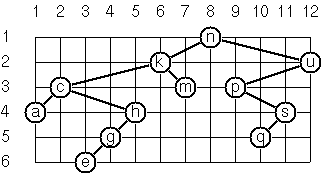In this layout strategy, the position of a node v is obtained by the following two rules:

• x(v) is equal to the position of the node v in the inorder sequence
• y(v) is equal to the depth of the node v in the tree

In order to store the position of the nodes, we extend the Prolog term representing a node (and its successors) as follows:

% nil represents the empty tree (as usual)
% t(W,X,Y,L,R) represents a (non-empty) binary tree with root W "positioned" at (X,Y), and subtrees L and R

Write a predicate layout_binary_tree/2 with the following specification:

% layout_binary_tree(T,PT) :- PT is the "positioned" binary tree obtained from the binary tree T. (+,?)

Test your predicate in an appropriate way.

P65 (**) Layout a binary tree (2)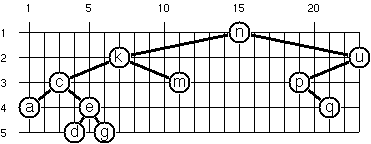An alternative layout method is depicted in the illustration opposite. Find out the rules and write the corresponding Prolog predicate. Hint: On a given level, the horizontal distance between neighboring nodes is constant.

Use the same conventions as in problem P64 and test your predicate in an appropriate way.

P66 (***) Layout a binary tree (3)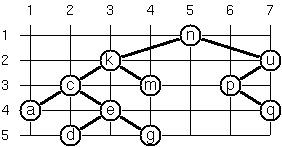Yet another layout strategy is shown in the illustration opposite. The method yields a very compact layout while maintaining a certain symmetry in every node. Find out the rules and write the corresponding Prolog predicate. Hint: Consider the horizontal distance between a node and its successor nodes. How tight can you pack together two subtrees to construct the combined binary tree?

Use the same conventions as in problem P64 and P65 and test your predicate in an appropriate way. Note: This is a difficult problem. Don't give up too early!

Which layout do you like most?

P67 (**) A string representation of binary treesSomebody represents binary trees as strings of the following type (see example opposite):

a(b(d,e),c(,f(g,)))

a) Write a Prolog predicate which generates this string representation, if the tree is given as usual (as nil or t(X,L,R) term). Then write a predicate which does this inverse; i.e. given the string representation, construct the tree in the usual form. Finally, combine the two predicates in a single predicate tree_string/2 which can be used in both directions.

b) Write the same predicate tree_string/2 using difference lists and a single predicate tree_dlist/2 which does the conversion between a tree and a difference list in both directions.

For simplicity, suppose the information in the nodes is a single letter and there are no spaces in the string.

P68 (**) Preorder and inorder sequences of binary trees
We consider binary trees with nodes that are identified by single lower-case letters, as in the example of problem P67.

a) Write predicates preorder/2 and inorder/2 that construct the preorder and inorder sequence of a given binary tree, respectively. The results should be atoms, e.g. 'abdecfg' for the preorder sequence of the example in problem P67.

b) Can you use preorder/2 from problem part a) in the reverse direction; i.e. given a preorder sequence, construct a corresponding tree? If not, make the necessary arrangements.

c) If both the preorder sequence and the inorder sequence of the nodes of a binary tree are given, then the tree is determined unambiguously. Write a predicate pre_in_tree/3 that does the job.

d) Solve problems a) to c) using difference lists. Cool! Use the predefined predicate time/1 to compare the solutions.

What happens if the same character appears in more than one node. Try for instance pre_in_tree(aba,baa,T).

P69 (**) Dotstring representation of binary trees
We consider again binary trees with nodes that are identified by single lower-case letters, as in the example of problem P67. Such a tree can be represented by the preorder sequence of its nodes in which dots (.) are inserted where an empty subtree (nil) is encountered during the tree traversal. For example, the tree shown in problem P67 is represented as 'abd..e..c.fg...'. First, try to establish a syntax (BNF or syntax diagrams) and then write a predicate tree_dotstring/2 which does the conversion in both directions. Use difference lists.

## Multiway Trees

A multiway tree is composed of a root element and a (possibly empty) set of successors which are multiway trees themselves. A multiway tree is never empty. The set of successor trees is sometimes called a forest.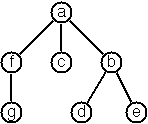In Prolog we represent a multiway tree by a term t(X,F), where X denotes the root node and F denotes the forest of successor trees (a Prolog list). The example tree depicted opposite is therefore represented by the following Prolog term:
```T = t(a,[t(f,[t(g,[])]),t(c,[]),t(b,[t(d,[]),t(e,[])])])
```

P70B (*) Check whether a given term represents a multiway tree
Write a predicate istree/1 which succeeds if and only if its argument is a Prolog term representing a multiway tree.
Example:
?- istree(t(a,[t(f,[t(g,[])]),t(c,[]),t(b,[t(d,[]),t(e,[])])])).
Yes

P70C (*) Count the nodes of a multiway tree
Write a predicate nnodes/1 which counts the nodes of a given multiway tree.
Example:
?- nnodes(t(a,[t(f,[])]),N).
N = 2

Write another version of the predicate that allows for a flow pattern (o,i).

P70 (**) Tree construction from a node stringWe suppose that the nodes of a multiway tree contain single characters. In the depth-first order sequence of its nodes, a special character ^ has been inserted whenever, during the tree traversal, the move is a backtrack to the previous level.

By this rule, the tree in the figure opposite is represented as: afg^^c^bd^e^^^

Define the syntax of the string and write a predicate tree(String,Tree) to construct the Tree when the String is given. Work with atoms (instead of strings). Make your predicate work in both directions.

P71 (*) Determine the internal path length of a tree
We define the internal path length of a multiway tree as the total sum of the path lengths from the root to all nodes of the tree. By this definition, the tree in the figure of problem P70 has an internal path length of 9. Write a predicate ipl(Tree,IPL) for the flow pattern (+,-).

P72 (*) Construct the bottom-up order sequence of the tree nodes
Write a predicate bottom_up(Tree,Seq) which constructs the bottom-up sequence of the nodes of the multiway tree Tree. Seq should be a Prolog list. What happens if you run your predicate backwords?

P73 (**) Lisp-like tree representation
There is a particular notation for multiway trees in Lisp. Lisp is a prominent functional programming language, which is used primarily for artificial intelligence problems. As such it is one of the main competitors of Prolog. In Lisp almost everything is a list, just as in Prolog everything is a term.

The following pictures show how multiway tree structures are represented in Lisp.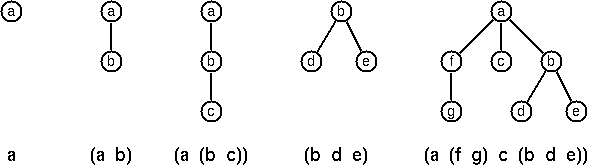Note that in the "lispy" notation a node with successors (children) in the tree is always the first element in a list, followed by its children. The "lispy" representation of a multiway tree is a sequence of atoms and parentheses '(' and ')', which we shall collectively call "tokens". We can represent this sequence of tokens as a Prolog list; e.g. the lispy expression (a (b c)) could be represented as the Prolog list ['(', a, '(', b, c, ')', ')']. Write a predicate tree_ltl(T,LTL) which constructs the "lispy token list" LTL if the tree is given as term T in the usual Prolog notation.

Example:
?- tree_ltl(t(a,[t(b,[]),t(c,[])]),LTL).
LTL = ['(', a, '(', b, c, ')', ')']

As a second, even more interesting exercise try to rewrite tree_ltl/2 in a way that the inverse conversion is also possible: Given the list LTL, construct the Prolog tree T. Use difference lists.

## Graphs

A graph is defined as a set of nodes and a set of edges, where each edge is a pair of nodes.

There are several ways to represent graphs in Prolog. One method is to represent each edge separately as one clause (fact). In this form, the graph depicted below is represented as the following predicate: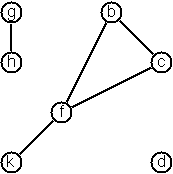```edge(h,g).
edge(k,f).
edge(f,b).
...
```
We call this edge-clause form. Obviously, isolated nodes cannot be represented. Another method is to represent the whole graph as one data object. According to the definition of the graph as a pair of two sets (nodes and edges), we may use the following Prolog term to represent the example graph:
```graph([b,c,d,f,g,h,k],[e(b,c),e(b,f),e(c,f),e(f,k),e(g,h)])
```
We call this graph-term form. Note, that the lists are kept sorted, they are really sets, without duplicated elements. Each edge appears only once in the edge list; i.e. an edge from a node x to another node y is represented as e(x,y), the term e(y,x) is not present. The graph-term form is our default representation. In SWI-Prolog there are predefined predicates to work with sets.

A third representation method is to associate with each node the set of nodes that are adjacent to that node. We call this the adjacency-list form. In our example:

```[n(b,[c,f]), n(c,[b,f]), n(d,[]), n(f,[b,c,k]), ...]
```
The representations we introduced so far are Prolog terms and therefore well suited for automated processing, but their syntax is not very user-friendly. Typing the terms by hand is cumbersome and error-prone. We can define a more compact and "human-friendly" notation as follows: A graph is represented by a list of atoms and terms of the type X-Y (i.e. functor '-' and arity 2). The atoms stand for isolated nodes, the X-Y terms describe edges. If an X appears as an endpoint of an edge, it is automatically defined as a node. Our example could be written as:
```[b-c, f-c, g-h, d, f-b, k-f, h-g]
```
We call this the human-friendly form. As the example shows, the list does not have to be sorted and may even contain the same edge multiple times. Notice the isolated node d. (Actually, isolated nodes do not even have to be atoms in the Prolog sense, they can be compound terms, as in d(3.75,blue) instead of d in the example).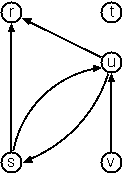When the edges are directed we call them arcs. These are represented by ordered pairs. Such a graph is called directed graph. To represent a directed graph, the forms discussed above are slightly modified. The example graph opposite is represented as follows:

Arc-clause form
arc(s,u).
arc(u,r).
...

Graph-term form
digraph([r,s,t,u,v],[a(s,r),a(s,u),a(u,r),a(u,s),a(v,u)])

[n(r,[]),n(s,[r,u]),n(t,[]),n(u,[r]),n(v,[u])]
Note that the adjacency-list does not have the information on whether it is a graph or a digraph.

Human-friendly form
[s > r, t, u > r, s > u, u > s, v > u]

Finally, graphs and digraphs may have additional information attached to nodes and edges (arcs). For the nodes, this is no problem, as we can easily replace the single character identifiers with arbitrary compound terms, such as city('London',4711). On the other hand, for edges we have to extend our notation. Graphs with additional information attached to edges are called labelled graphs.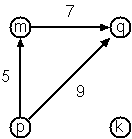Arc-clause form
arc(m,q,7).
arc(p,q,9).
arc(p,m,5).

Graph-term form
digraph([k,m,p,q],[a(m,p,7),a(p,m,5),a(p,q,9)])

[n(k,[]),n(m,[q/7]),n(p,[m/5,q/9]),n(q,[])]
Notice how the edge information has been packed into a term with functor '/' and arity 2, together with the corresponding node.

Human-friendly form
[p>q/9, m>q/7, k, p>m/5]

The notation for labelled graphs can also be used for so-called multi-graphs, where more than one edge (or arc) are allowed between two given nodes.

P80 (***) Conversions
Write predicates to convert between the different graph representations. With these predicates, all representations are equivalent; i.e. for the following problems you can always pick freely the most convenient form. The reason this problem is rated (***) is not because it's particularly difficult, but because it's a lot of work to deal with all the special cases.

P81 (**) Path from one node to another one
Write a predicate path(G,A,B,P) to find an acyclic path P from node A to node b in the graph G. The predicate should return all paths via backtracking.

P82 (*) Cycle from a given node
Write a predicate cycle(G,A,P) to find a closed path (cycle) P starting at a given node A in the graph G. The predicate should return all cycles via backtracking.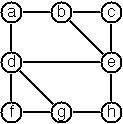P83 (**) Construct all spanning trees
Write a predicate s_tree(Graph,Tree) to construct (by backtracking) all spanning trees of a given graph. With this predicate, find out how many spanning trees there are for the graph depicted to the left. The data of this example graph can be found in the file p83.dat. When you have a correct solution for the s_tree/2 predicate, use it to define two other useful predicates: is_tree(Graph) and is_connected(Graph). Both are five-minutes tasks!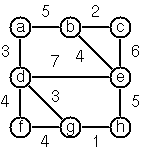P84 (**) Construct the minimal spanning tree
Write a predicate ms_tree(Graph,Tree,Sum) to construct the minimal spanning tree of a given labelled graph. Hint: Use the algorithm of Prim. A small modification of the solution of P83 does the trick. The data of the example graph to the right can be found in the file p84.dat.

P85 (**) Graph isomorphism
Two graphs G1(N1,E1) and G2(N2,E2) are isomorphic if there is a bijection f: N1 -> N2 such that for any nodes X,Y of N1, X and Y are adjacent if and only if f(X) and f(Y) are adjacent.

Write a predicate that determines whether two graphs are isomorphic. Hint: Use an open-ended list to represent the function f.

P86 (**) Node degree and graph coloration
a) Write a predicate degree(Graph,Node,Deg) that determines the degree of a given node.

b) Write a predicate that generates a list of all nodes of a graph sorted according to decreasing degree.

c) Use Welch-Powell's algorithm to paint the nodes of a graph in such a way that adjacent nodes have different colors.

P87 (**) Depth-first order graph traversal (alternative solution)
Write a predicate that generates a depth-first order graph traversal sequence. The starting point should be specified, and the output should be a list of nodes that are reachable from this starting point (in depth-first order).

P88 (**) Connected components (alternative solution)
Write a predicate that splits a graph into its connected components.

P89 (**) Bipartite graphs
Write a predicate that finds out whether a given graph is bipartite.

## Miscellaneous Problems

P90 (**) Eight queens problem
This is a classical problem in computer science. The objective is to place eight queens on a chessboard so that no two queens are attacking each other; i.e., no two queens are in the same row, the same column, or on the same diagonal.

Hint: Represent the positions of the queens as a list of numbers 1..N. Example: [4,2,7,3,6,8,5,1] means that the queen in the first column is in row 4, the queen in the second column is in row 2, etc. Use the generate-and-test paradigm.

P91 (**) Knight's tour
Another famous problem is this one: How can a knight jump on an NxN chessboard in such a way that it visits every square exactly once?

Hints: Represent the squares by pairs of their coordinates of the form X/Y, where both X and Y are integers between 1 and N. (Note that '/' is just a convenient functor, not division!) Define the relation jump(N,X/Y,U/V) to express the fact that a knight can jump from X/Y to U/V on a NxN chessboard. And finally, represent the solution of our problem as a list of N*N knight positions (the knight's tour).

P92 (***) Von Koch's conjecture
Several years ago I met a mathematician who was intrigued by a problem for which he didn't know a solution. His name was Von Koch, and I don't know whether the problem has been solved since.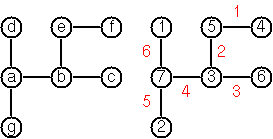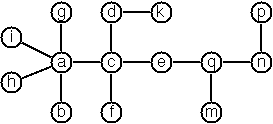Anyway the puzzle goes like this: Given a tree with N nodes (and hence N-1 edges). Find a way to enumerate the nodes from 1 to N and, accordingly, the edges from 1 to N-1 in such a way, that for each edge K the difference of its node numbers equals to K. The conjecture is that this is always possible.

For small trees the problem is easy to solve by hand. However, for larger trees, and 14 is already very large, it is extremely difficult to find a solution. And remember, we don't know for sure whether there is always a solution!

Write a predicate that calculates a numbering scheme for a given tree. What is the solution for the larger tree pictured above?

P93 (***) An arithmetic puzzle
Given a list of integer numbers, find a correct way of inserting arithmetic signs (operators) such that the result is a correct equation. Example: With the list of numbers [2,3,5,7,11] we can form the equations 2-3+5+7 = 11 or 2 = (3*5+7)/11 (and ten others!).

P94 (***) Generate K-regular simple graphs with N nodes
In a K-regular graph all nodes have a degree of K; i.e. the number of edges incident in each node is K. How many (non-isomorphic!) 3-regular graphs with 6 nodes are there? See also a table of results and a Java applet that can represent graphs geometrically.

P95 (**) English number words
On financial documents, like cheques, numbers must sometimes be written in full words. Example: 175 must be written as one-seven-five. Write a predicate full_words/1 to print (non-negative) integer numbers in full words.

P96 (**) Syntax checker (alternative solution with difference lists)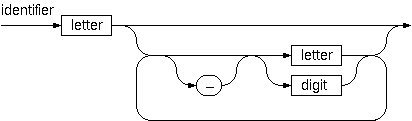In a certain programming language (Ada) identifiers are defined by the syntax diagram (railroad chart) opposite. Transform the syntax diagram into a system of syntax diagrams which do not contain loops; i.e. which are purely recursive. Using these modified diagrams, write a predicate identifier/1 that can check whether or not a given string is a legal identifier.

% identifier(Str) :- Str is a legal identifier
P97 (**) Sudoku
Sudoku puzzles go like this:
```   Problem statement                 Solution

.  .  4 | 8  .  . | .  1  7	     9  3  4 | 8  2  5 | 6  1  7
|         |                      |         |
6  7  . | 9  .  . | .  .  .	     6  7  2 | 9  1  4 | 8  5  3
|         |                      |         |
5  .  8 | .  3  . | .  .  4      5  1  8 | 6  3  7 | 9  2  4
--------+---------+--------      --------+---------+--------
3  .  . | 7  4  . | 1  .  .      3  2  5 | 7  4  8 | 1  6  9
|         |                      |         |
.  6  9 | .  .  . | 7  8  .      4  6  9 | 1  5  3 | 7  8  2
|         |                      |         |
.  .  1 | .  6  9 | .  .  5      7  8  1 | 2  6  9 | 4  3  5
--------+---------+--------      --------+---------+--------
1  .  . | .  8  . | 3  .  6	     1  9  7 | 5  8  2 | 3  4  6
|         |                      |         |
.  .  . | .  .  6 | .  9  1	     8  5  3 | 4  7  6 | 2  9  1
|         |                      |         |
2  4  . | .  .  1 | 5  .  .      2  4  6 | 3  9  1 | 5  7  8
```

Every spot in the puzzle belongs to a (horizontal) row and a (vertical) column, as well as to one single 3x3 square (which we call "square" for short). At the beginning, some of the spots carry a single-digit number between 1 and 9. The problem is to fill the missing spots with digits in such a way that every number between 1 and 9 appears exactly once in each row, in each column, and in each square.

P98 (***) Nonograms
Around 1994, a certain kind of puzzles was very popular in England. The "Sunday Telegraph" newspaper wrote: "Nonograms are puzzles from Japan and are currently published each week only in The Sunday Telegraph. Simply use your logic and skill to complete the grid and reveal a picture or diagram." As a Prolog programmer, you are in a better situation: you can have your computer do the work! Just write a little program ;-).

The puzzle goes like this: Essentially, each row and column of a rectangular bitmap is annotated with the respective lengths of its distinct strings of occupied cells. The person who solves the puzzle must complete the bitmap given only these lengths.

```          Problem statement:          Solution:

|_|_|_|_|_|_|_|_| 3         |_|X|X|X|_|_|_|_| 3
|_|_|_|_|_|_|_|_| 2 1       |X|X|_|X|_|_|_|_| 2 1
|_|_|_|_|_|_|_|_| 3 2       |_|X|X|X|_|_|X|X| 3 2
|_|_|_|_|_|_|_|_| 2 2       |_|_|X|X|_|_|X|X| 2 2
|_|_|_|_|_|_|_|_| 6         |_|_|X|X|X|X|X|X| 6
|_|_|_|_|_|_|_|_| 1 5       |X|_|X|X|X|X|X|_| 1 5
|_|_|_|_|_|_|_|_| 6         |X|X|X|X|X|X|_|_| 6
|_|_|_|_|_|_|_|_| 1         |_|_|_|_|X|_|_|_| 1
|_|_|_|_|_|_|_|_| 2         |_|_|_|X|X|_|_|_| 2
1 3 1 7 5 3 4 3             1 3 1 7 5 3 4 3
2 1 5 1                     2 1 5 1
```
For the example above, the problem can be stated as the two lists [,[2,1],[3,2],[2,2],,[1,5],,,] and [[1,2],[3,1],[1,5],[7,1],,,,] which give the "solid" lengths of the rows and columns, top-to-bottom and left-to-right, respectively. Published puzzles are larger than this example, e.g. 25 x 20, and apparently always have unique solutions.

P99 (***) Crossword puzzle
Given an empty (or almost empty) framework of a crossword puzzle and a set of words. The problem is to place the words into the framework.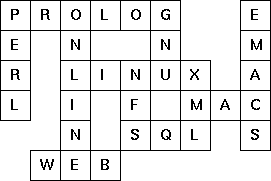The particular crossword puzzle is specified in a text file which first lists the words (one word per line) in an arbitrary order. Then, after an empty line, the crossword framework is defined. In this framework specification, an empty character location is represented by a dot (.). In order to make the solution easier, character locations can also contain predefined character values. The puzzle opposite is defined in the file p99a.dat, other examples are p99b.dat and p99d.dat. There is also an example of a puzzle (p99c.dat) which does not have a solution.

Words are strings (character lists) of at least two characters. A horizontal or vertical sequence of character places in the crossword puzzle framework is called a site. Our problem is to find a compatible way of placing words onto sites.

Hints: (1) The problem is not easy. You will need some time to thoroughly understand it. So, don't give up too early! And remember that the objective is a clean solution, not just a quick-and-dirty hack!
(2) Reading the data file is a tricky problem for which a solution is provided in the file p99-readfile.pl. Use the predicate read_lines/2.
(3) For efficiency reasons it is important, at least for larger puzzles, to sort the words and the sites in a particular order. For this part of the problem, the solution of P28 may be very helpful.